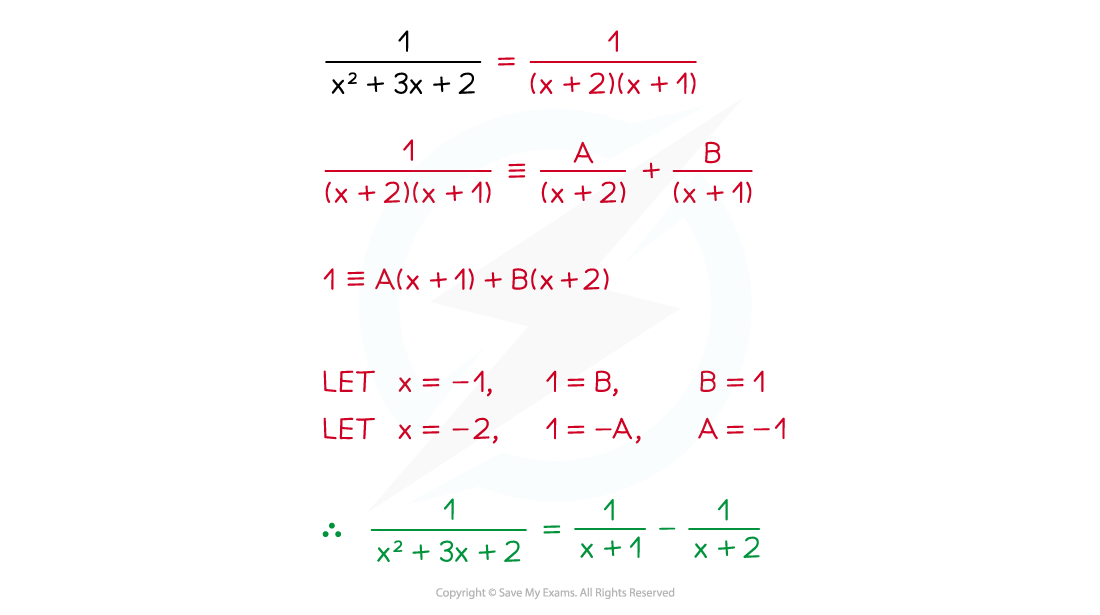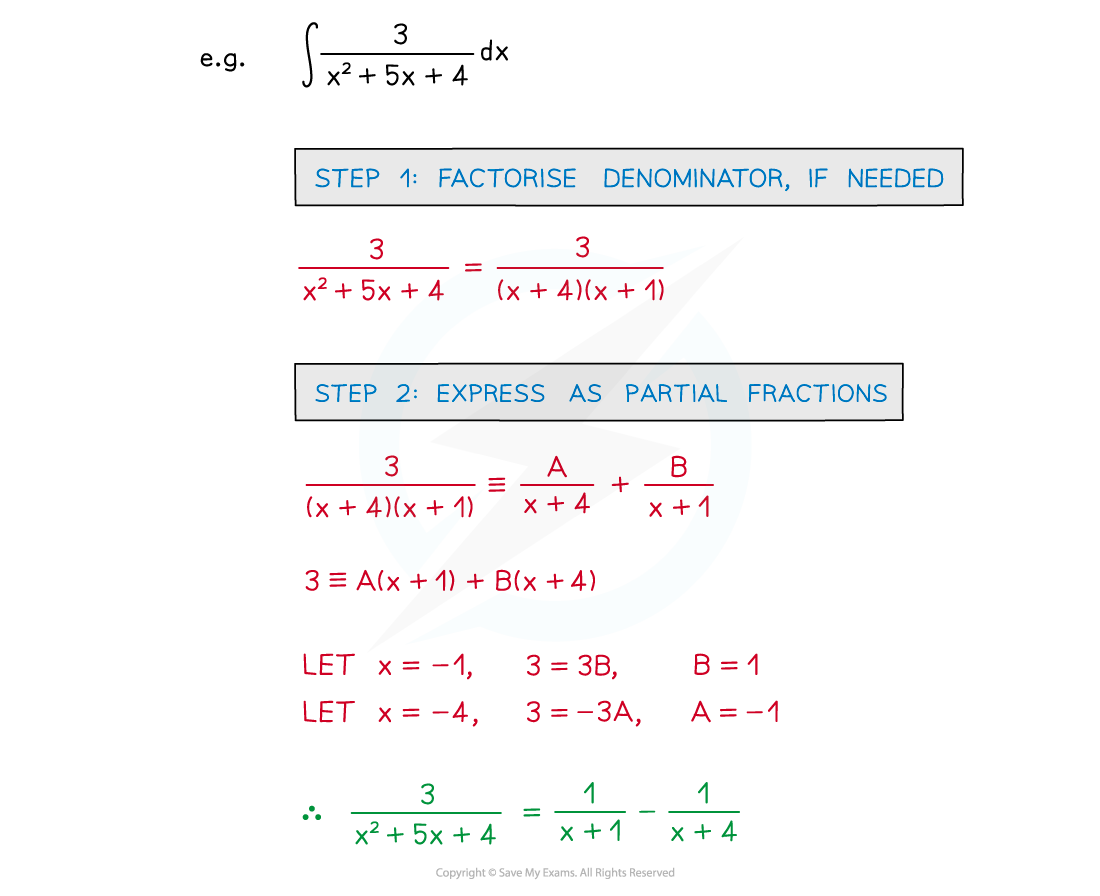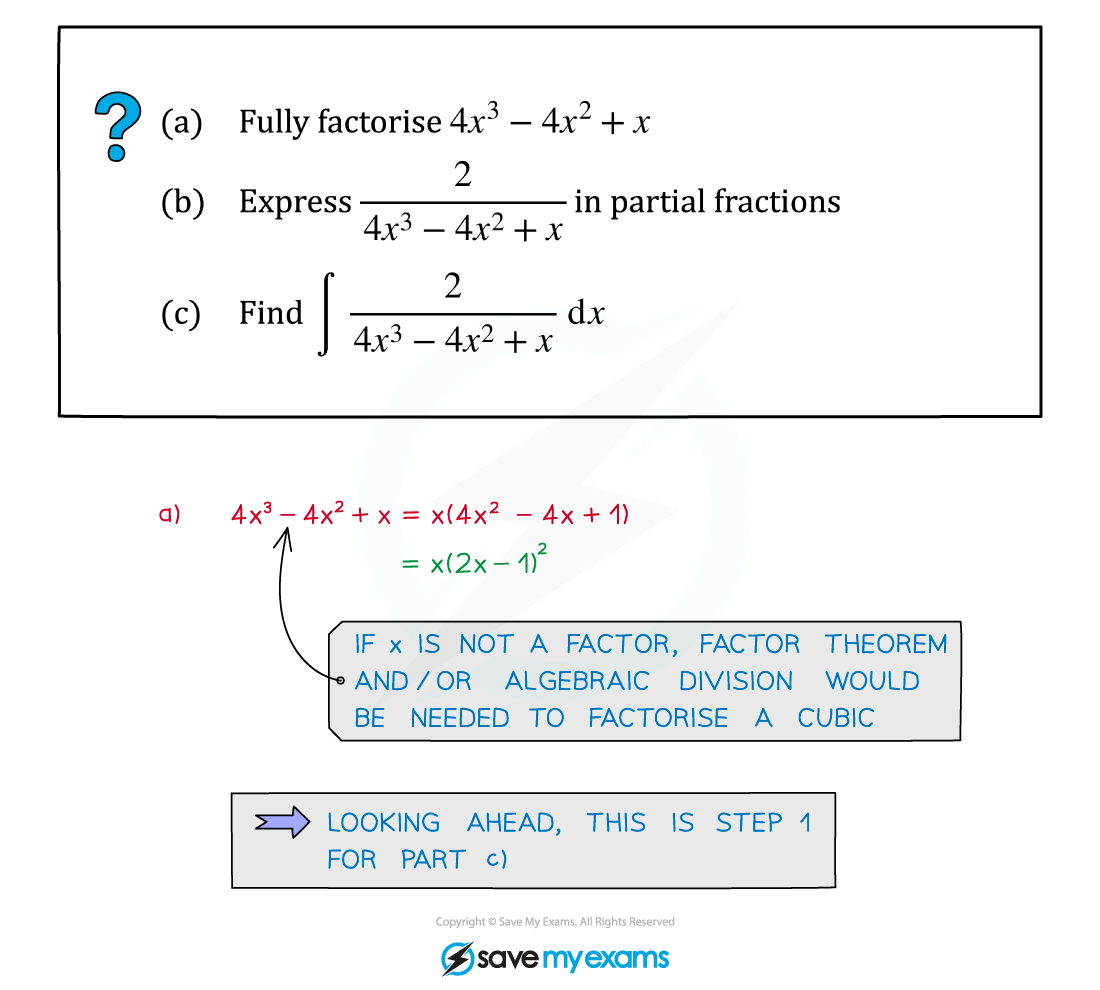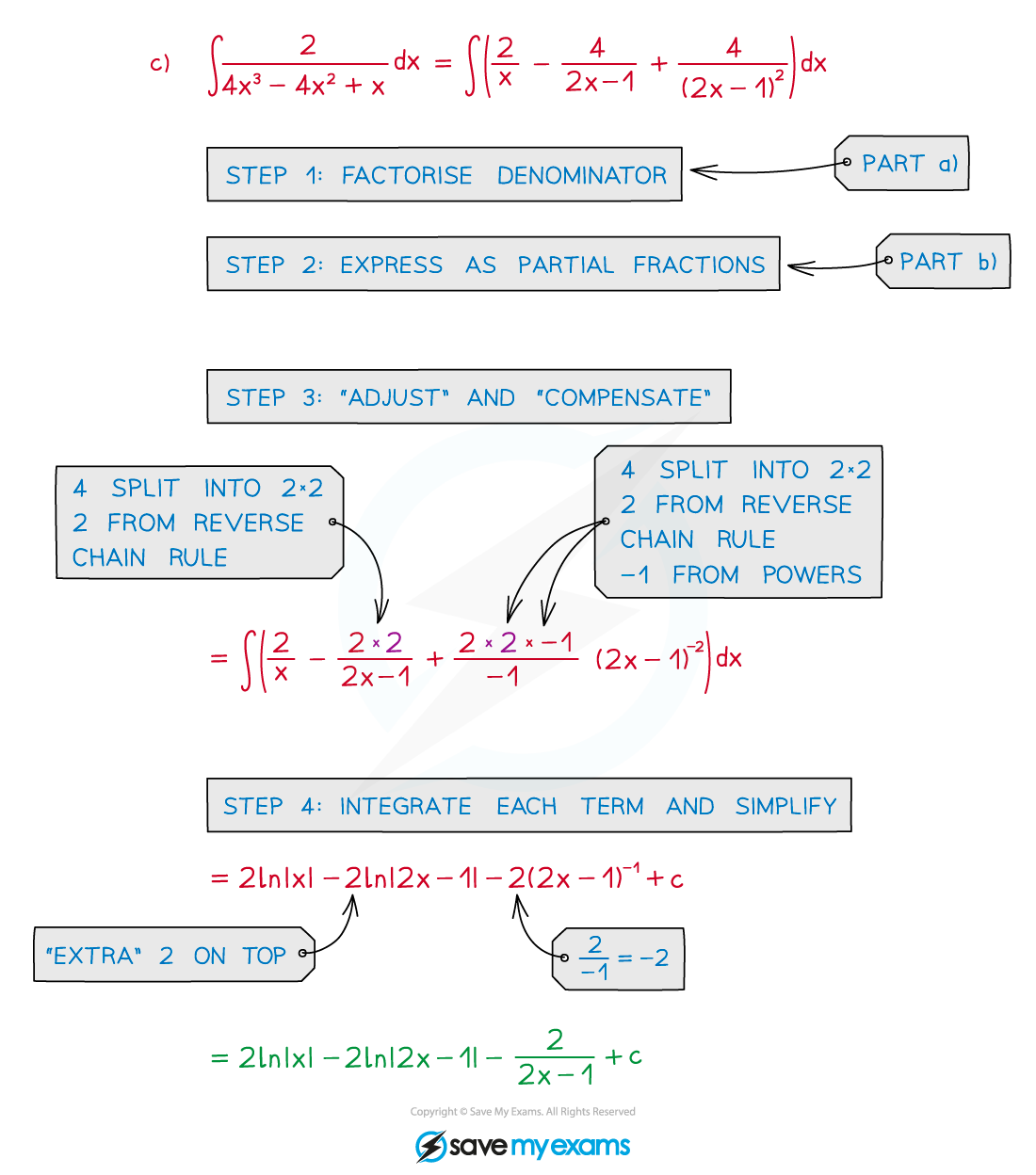# CIE A Level Maths: Pure 3复习笔记5.1.7 Integration using Partial Fractions

### Integration using Partial Fractions

#### What are Partial Fractions?

• This is the reverse process to adding (or subtracting) fractions
• The common polynomial denominator is split into factors
• Make sure you are familiar with the notes on Partial Fractions#### How do I integrate using partial fractions?

• Fractions with linear denominators can be integrated (See Integrating Other Functions)• A fraction with a polynomial denominator (degree 2+) can be integrated by …
• … splitting using partial fractions
• … integrating each partial fraction• STEP 1: Factorise denominator, if needed
• STEP 2: Express as partial fractions
• STEP 3: ‘Adjust’ and ‘compensate’ each term so it can be integrated (See Reverse Chain Rule)
• STEP 4: Integrate each term (usually involves ln) and simplify

#### Exam Tip

• When there are several parts, read the whole question:
• An early part may be a “show that” involving partial fractions
• A later part may be to integrate the original fraction
• If you can’t do, or get stuck on, the partial fractions bit of the question you can still use the “show that” result to help with the integration.

#### Worked Example